Download Presentation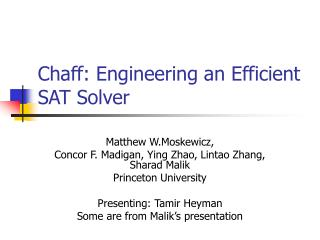Chaff: Engineering an Efficient SAT Solver

# Chaff: Engineering an Efficient SAT Solver - PowerPoint PPT Presentation

Chaff: Engineering an Efficient SAT Solver. Matthew W.Moskewicz, Concor F. Madigan, Ying Zhao, Lintao Zhang, Sharad Malik Princeton University Presenting: Tamir Heyman Some are from Malik’s presentation. Chaff Philosophy. Make the core operations fastI am the owner, or an agent authorized to act on behalf of the owner, of the copyrighted work described.
Download Presentation## Chaff: Engineering an Efficient SAT Solver

An Image/Link below is provided (as is) to download presentation

Download Policy: Content on the Website is provided to you AS IS for your information and personal use and may not be sold / licensed / shared on other websites without getting consent from its author.While downloading, if for some reason you are not able to download a presentation, the publisher may have deleted the file from their server.

- - - - - - - - - - - - - - - - - - - - - - - - - - E N D - - - - - - - - - - - - - - - - - - - - - - - - - -
Presentation Transcript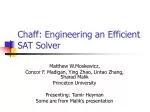### Chaff: Engineering an Efficient SAT Solver

Matthew W.Moskewicz,

Concor F. Madigan, Ying Zhao, Lintao Zhang, Sharad Malik

Princeton University

Presenting: Tamir Heyman

Some are from Malik’s presentationChaff Philosophy
• Make the core operations fast
• profiling driven, most time-consuming parts:
• Boolean Constraint Propagation (BCP) and Decision
• Emphasis on coding efficiency
• Emphasis on optimizing data cache behavior
• As always, good search space pruning (i.e. conflict resolution and learning) is importantChaff’s Main Procedures
• Efficient BCP
• Two watched literals
• Fast back tracking
• Efficient Decision procedure
• Localizes search space
• Restart
• Increases robustness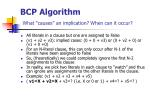• All literals in a clause but one are assigned to False
• (v1 + v2 + v3): implied cases: (0 + 0 + v3) or (0 + v2 + 0) or (v1 + 0 +0)
• For an N-literal clause, this can only occur after N-1 of the literals have been assigned to False
• So, (theoretically) we could completely ignore the first N-2 assignments to this clause
• In reality, we pick two literals in each clause to “watch” and thus can ignore any assignments to the other literals in the clause.
• Example: (v1 + v2 + v3 + v4 + v5)
• ( v1=X + v2=X + v3=? {i.e. X or 0 or 1} + v4=? + v5=? )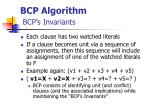BCP AlgorithmBCP’s Invariants
• Each clause has two watched literals
• If a clause becomes unit via a sequence of assignments, then this sequence will include an assignment of one of the watched literals to F
• Example again: (v1 + v2 + v3 + v4 + v5)
• ( v1=X + v2=X + v3=? + v4=? + v5=? )
• BCP consists of identifying unit (and conflict) clauses (and the associated implications) while maintaining the “BCP’s Invariants”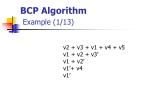BCP AlgorithmExample (1/13)

v2 + v3 + v1 + v4 + v5

v1 + v2 + v3’

v1 + v2’

v1’+ v4

v1’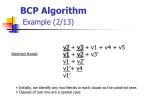BCP AlgorithmExample (2/13)

v2+ v3 + v1 + v4 + v5

v1 + v2 + v3’

v1 + v2’

v1’+ v4

v1’

Watched literals

• Initially, we identify any two literals in each clause as the watched ones
• Clauses of size one are a special case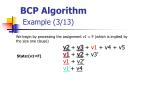BCP AlgorithmExample (3/13)

We begin by processing the assignment v1 = F (which is implied by

the size one clause)

v2+ v3 + v1 + v4 + v5

v1 + v2 + v3’

v1 + v2’

v1’+ v4

State:(v1=F)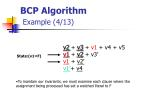BCP AlgorithmExample (4/13)

v2+ v3 + v1 + v4 + v5

v1 + v2 + v3’

v1 + v2’

v1’+ v4

State:(v1=F)

• To maintain our invariants, we must examine each clause where the
• assignment being processed has set a watched literal to F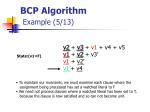BCP AlgorithmExample (5/13)

v2+ v3 + v1 + v4 + v5

v1 + v2 + v3’

v1 + v2’

v1’+ v4

State:(v1=F)

• To maintain our invariants, we must examine each clause where the
• assignment being processed has set a watched literal to F
• We need not process clauses where a watched literal has been set to T,
• because the clause is now satisfied and so can not become unit.BCP AlgorithmExample (6/13)

v2+ v3 + v1 + v4 + v5

v1 + v2 + v3’

v1 + v2’

v1’+ v4

State:(v1=F)

• To maintain our invariants, we must examine each clause where the
• assignment being processed has set a watched literal to F
• We need not process clauses where a watched literal has been set to T,
• because the clause is now satisfied and so can not become unit.
• We certainly need not process any clauses where neither watch
• literal changes state (in this example, where v1 is not watched).BCP AlgorithmExample (7/13)
• Now let’s actually process the second and third clauses:

v2+ v3 + v1 + v4 + v5

v1 + v2 + v3’

v1 + v2’

v1’+ v4

State:(v1=F)BCP AlgorithmExample (8/13)
• Now let’s actually process the second and third clauses:

v2+ v3 + v1 + v4 + v5

v1 + v2 + v3’

v1 + v2’

v1’+ v4

v2+ v3 + v1 + v4 + v5

v1 + v2 + v3’

v1 + v2’

v1’+ v4

State:(v1=F)

State:(v1=F)

• For the second clause, we replace v1 with v3’ as a new watched literal.
• Since v3’ is not assigned to F, this maintains our invariants.BCP AlgorithmExample (9/13)
• Now let’s actually process the second and third clauses:

v2+ v3 + v1 + v4 + v5

v1 + v2 + v3’

v1 + v2’

v1’+ v4

v2+ v3 + v1 + v4 + v5

v1 + v2 + v3’

v1 + v2’

v1’+ v4

State:(v1=F)

Pending: (v2=F)

State:(v1=F)

• For the second clause, we replace v1 with v3’ as a new watched literal.
• Since v3’ is not assigned to F, this maintains our invariants.
• The third clause is unit. We record the new implication of v2’, and add it to the
• queue of assignments to process. Since the clause cannot again become unit,
• our invariants are maintained.BCP AlgorithmExample (10/13)
• Next, we process v2’. We only examine the first 2 clauses

v2 + v3 + v1 + v4 + v5

v1 + v2+ v3’

v1 + v2’

v1’+ v4

V2 + v3 + v1 + v4 + v5

v1 + v2 + v3’

v1 + v2’

v1’+ v4

State:(v1=F, v2=F)

Pending: (v3=F)

State:(v1=F, v2=F)

In the first clause, we replace v2 with v4 as a new watched literal. Since v4 is not assigned to F, this maintains our invariants.

The second clause is unit. We record the new implication of v3’, and add it to the queue of assignments to process. Since the clause cannot again become unit, our invariants are maintainedBCP AlgorithmExample (11/13)
• Next, we process v3’. We only examine the first clause.

v2 + v3 + v1 + v4 + v5

v1 + v2+ v3’

v1 + v2’

v1’+ v4

V2 + v3 + v1 + v4 + v5

v1 + v2 + v3’

v1 + v2’

v1’+ v4

State:(v1=F, v2=F, v3=F)

Pending:

State:(v1=F, v2=F, v3=F)

For the first clause, we replace v3 with v5 as a new watched literal. Since v5 is not assigned to F, this maintains our invariants.

Since there are no pending assignments, and no conflict, BCP terminates and we make a decision. Both v4 and v5 are unassigned. Let’s say we decide to assign v4=T and proceed.BCP AlgorithmExample (12/13)
• Next, we process v4. We do nothing at all.

v2 + v3 + v1 + v4 + v5

v1 + v2+ v3’

v1 + v2’

v1’+ v4

V2 + v3 + v1 + v4 + v5

v1 + v2 + v3’

v1 + v2’

v1’+ v4

State:(v1=F, v2=F, v3=F , v4=T)

Pending:

State:(v1=F, v2=F, v3=F, v4=T)

Since there are no pending assignments, and no conflict, BCP terminates and we make a decision. Only v5 is unassigned. Let’s say we decide to assign v5=F and proceedBCP AlgorithmExample (13/13)
• Next, we process v5=F

v2 + v3 + v1 + v4 + v5

v1 + v2+ v3’

v1 + v2’

v1’+ v4

V2 + v3 + v1 + v4 + v5

v1 + v2 + v3’

v1 + v2’

v1’+ v4

State:(v1=F, v2=F, v3=F, v4=T, v5=F)

State:(v1=F, v2=F, v3=F , v4=T , v5=F)

The first clause is already satisfied by v4 so we ignore it.

Since there are no pending assignments, and no conflict, BCP terminates and we make a decision. No variables are unassigned, so the instance is SAT, and we are done.BCP Algorithm Summary
• During forward progress: Decisions and Implications
• Only need to examine clauses where watched literal is set to F
• Can ignore any assignments of literals to T
• Can ignore any assignments to non-watched literals
• During backtrack: Unwind Assignment Stack
• Any sequence of chronological unassignments will maintain our invariants
• So no action is required at all to unassign variables.
• Overall
• Minimize clause accessChaff Decision HeuristicVSIDS
• Variable State Independent Decaying Sum
• Rank variables by literal count in the initial clause database
• Only increment counts as new clauses are added.
• Periodically, divide all counts by a constant.VSIDS Example (1/3)

Initial data base

x1 + x4

x1 + x3’ + x8’

x1 + x8 + x12

x2 + x11

x7’ + x3’ + x9

x7’ + x8 + x9’

x7 + x8 + x10’

Scores:

4: x8

3: x1,x7

2: x3

1: x2,x4,x9,x10,x11,x12

x1 + x4

x1 + x3’ + x8’

x1 + x8 + x12

x2 + x11

x7’ + x3’ + x9

x7’ + x8 + x9’

x7 + x8 + x10’

x7 + x10 + x12’

Scores:

4: x8,x7

3: x1

2: x3,x10,x12

1: x2,x4,x9,x11VSIDS Example (2/3)

Counters divided by 2

x1 + x4

x1 + x3’ + x8’

x1 + x8 + x12

x2 + x11

x7’ + x3’ + x9

x7’ + x8 + x9’

x7 + x8 + x10’

x7 + x10 + x12’

Scores:

2: x8,x7, x1

1: x3,x10,x12

0: x2,x4,x9,x11VSIDS Example (3/3)

Counters divided by 2 before new clause added

x1 + x4

x1 + x3’ + x8’

x1 + x8 + x12

x2 + x11

x7’ + x3’ + x9

x7’ + x8 + x9’

x7 + x8 + x10’

x7 + x10 + x12’

Scores:

3: x7

2: x8,x7

1: x3,x10,x12

0: x2,x4,x9,x11Chaff Decision HeuristicVSIDS - Summary
• Quasi-static:
• Static because it doesn’t depend on variable state
• Not static because it gradually changes as new clauses are added
• Decay causes bias toward *recent* conflicts.
• Use heap to find unassigned variable with the highest ranking
• Even single linear pass though variables on each decision would dominate run-time!Interplay of BCP and the Decision Heuristic
• This is only an intuitive description …
• Reality depends heavily on specific instance
• Take some variable ranking (from the decision engine)
• Assume several decisions are made
• Say v2=T, v7=F, v9=T, v1=T (and any implications thereof)
• Then a conflict is encountered that forces v2=F
• The next decisions may still be v7=F, v9=T, v1=T !
• VSIDS variable ranks change slowly…
• But the BCP engine has recently processed these assignments …
• so these variables are unlikely to still be watched.
• In a more general sense, the more “active” a variable is, the more likely it is to *not* be watched.Interplay of Learning and the Decision Heuristic
• Again, this is an intuitive description …
• Learnt clauses capture relationships between variables
• Learnt clauses bias decision strategy to a smaller set of variables through decision heuristics like VSIDS
• Important when there are 100k variables!
• Decision heuristic influences which variables appear in learnt clauses
• Decisions →implications →conflicts →learnt clause
• Important for decisions to keep search strongly localizedRestart
• Abandon the current search tree and reconstruct a new one
• Helps reduce variance - adds to robustness in the solver
• The clauses learned prior to the restart are still there after the restart and can help pruning the search spaceTimeLine

1996

SATO

1k var

1960

DP

10 var

1988

SOCRATES

 3k var

1994

Hannibal

 3k var

1996

GRASP

1k var

1996

Stålmarck

 1000 var

1962

DLL

 10 var

1986

BDD

 100 var

1992

GSAT

 300 var

2001

Chaff

10k var